### Ryan A. Chisholm and Mark A. Burgman. 2004. The unified neutral theory of biodiversity and biogeography: comment. Ecology 85:3172–3174.

Appendix A. Metacommunity dynamics and neutral ecology.

Neutral ecology was studied by both Hubbell (2001) and Volkov et al. (2003) in the context of metacommunity models undergoing zero-sum dynamics. However, whereas Hubbell’s metacommunity was a non-overlapping generations model, Volkov et al. used an overlapping generations model, and while Hubbell’s relative species abundance (RSA) distribution was Fisher’s logarithmic series (log-series) (Fisher et al. 1943) only in the limit as the community size JM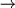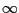, the RSA distribution of Volkov et al. is Fisher’s log-series for all values of JM.  Fisher’s log-series is defined as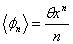, (A.1)

where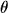(originally referred to by Fisher as) is a measure of diversity, 0 < x < 1, and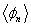is the frequency of species containing n individuals in the metacommunity.  Using the basic properties of series one can derive the expressions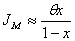(A.2) and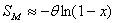, (A.3)

which are very close approximations to the number of individuals and the number of species in the metacommunity.  The error in (A.2) is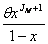while the error in (A.3) is readily shown to be less than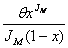, both of which are very small quantities.

In Hubbell’s Unified Neutral Theory of Biodiversity and Biogeographyis defined as the “fundamental biodiversity number” (Hubbell 2001)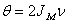. (A.4)

By substituting Eq. A.4 into Eq. A.2, it is then apparent that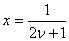. (A.5)

The number of species in the metacommunity is then given by substituting Eqs. A.4 and A.5 into Eq. A.3: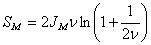. (A.6)

If we then set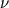= 3.8147 × 10-4 and JM = 65536, we obtain= 50 and SM = 358.95.  That is, there are approximately 359 species in Hubbell’s metacommunity at equilibrium.  This result is confirmed by simulations.

The metacommunity model of Volkov et al. (2003) is a time evolution problem with overlapping generations where pn,k(t) denotes the probability that the kth species contains n individuals at time t. The steady-state solution to the problem is then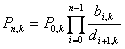(A.7)

for n > 0, where bn,k and dn,k denote the probability of birth and death, respectively, in the kth species with n individuals, and b-1,k = d0,k = 0 (Volkov et al. 2003). In neutral ecology, where all species are competitively equal, Eq. A.7 reduces to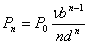(A.8)

where b and d are the probabilities of a random individual in the metacommunity giving birth and dying respectively,is a non-zero probability of speciation, and Pn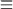Pn,kP0 can then be determined by normalization condition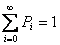(Volkov et al. 2003). A concise analytical expression for P0 can be obtained as follows:. (A.9)

Of course, for a finite-size metacommunity the probability Pn that a species has n individuals at any given time, is only non-zero for n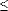JM. As such, for a real metacommunity, Expression A.9 represents only a close approximation.

In the log-series distribution for metacommunity RSA that Volkov et al. derived, the parametersand x were shown to be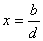(A.10)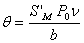(A.11)

where S'M is the number of species in the metacommunity (Volkov et al. 2003) including those with zero individuals.  The relationship between SM and S'M is simply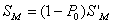. (A.12)

Under zero-sum dynamics in neutral ecology the probability d of a random individual dying is simply 1/JM. Following a death, a new individual will be generated by a speciation event with probability v, or by a birth with probability 1 –. The unconditional probability b of a random individual in the metacommunity giving birth is then (1 –)/JM. Substituting these expressions for b and d into Eqs. A.9, A.10 and A.11 yields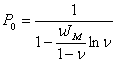(A.13)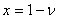(A.14)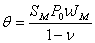(A.15)

By substituting Eq. A.14 into Eq. A.2 and rearranging, we obtain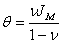. (A.16)

We can now find the expected values ofand SM under the overlapping generations model of Volkov et al. for the same values= 3.8147 – 10-4 and JM = 65536 as used in Hubbell’s model earlier. From Eq. A.14 we obtain x = 0.99962, and from Eq. A.16 we obtain= 25.0095. From Eq. A.13 we obtain P0 = 0.005054, and by substituting this into Eq. A.11 and rearranging, we then obtain S'M = 197.86. Equation A.12 then gives SM = 196.86. Alternatively SM can be determined from Eq. A.3, which again gives SM = 196.86. Thus there will be approximately 197 species in the metacommunity of Volkov et al. at equilibrium. This result is also confirmed by simulations.

From Eqs. A.4 and A.16 it can be seen that for similar values ofand JM, the fundamental biodiversity number of Volkov et al. will always be approximately half that of Hubbell’s providing thatis small. The total number of species predicted by the two models also differs significantly. For most ecological applications an overlapping generations model is more realistic, and as such the model of Volkov et al. may be preferable. In certain circumstances, such as a community of annual plants, Hubbell’s non-overlapping generations model may be more appropriate.

LITERATURE CITED

Fisher, R. A., A. S. Corbet, and C. B. Williams. 1943. The relation between the number of species and the number of individuals in a random sample of an animal population. Journal of Animal Ecology 12:42–58.

Hubbell, S. P. 2001. The unified neutral theory of biodiversity and biogeography. Princeton University Press, Princeton, New Jersey, USA.

Volkov, I., J. R. Banavar, S. P. Hubbell, and A. Maritan. 2003. Neutral theory and relative species abundance in ecology. Nature 424:1035–1037.

[Back to E085-105]